Symbolic gradient is usually computed from gradient.grad(), which offers a more convenient syntax for the common case of wanting the gradient of some scalar cost with respect to some input expressions. The grad_sources_inputs() function does the underlying work, and is more flexible, but is also more awkward to use when gradient.grad() can do the job.

Construct a graph for the gradient with respect to each input variable.

Each returned Variable represents the gradient with respect to that input computed based on the symbolic gradients with respect to each output. If the output is not differentiable with respect to an input, then this method should return an instance of type NullType for that input.

Using the reverse-mode AD characterization given in _, for a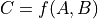representing the function implemented by the Op and its two arguments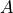and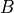, given by the Variables in inputs, the values returned by Op.grad represent the quantities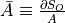and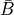, for some scalar output term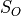of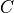in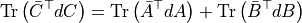Parameters:
• inputs – The input variables.

Returns:

• grads – The gradients with respect to each Variable in inputs.

• ..  Giles, Mike. 2008. “An Extended Collection of Matrix Derivative Results for Forward and Reverse Mode Automatic Differentiation.”

R_op(inputs, eval_points)[source]#

Construct a graph for the R-operator.

This method is primarily used by Rop.

Parameters:
• inputs – The Op inputs.

• eval_points – A Variable or list of Variables with the same length as inputs. Each element of eval_points specifies the value of the corresponding input at the point where the R-operator is to be evaluated.

Return type:

rval[i] should be Rop(f=f_i(inputs), wrt=inputs, eval_points=eval_points).

Construct a graph for the gradient with respect to each input variable.

Each returned Variable represents the gradient with respect to that input computed based on the symbolic gradients with respect to each output. If the output is not differentiable with respect to an input, then this method should return an instance of type NullType for that input.

Using the reverse-mode AD characterization given in _, for arepresenting the function implemented by the Op and its two argumentsand, given by the Variables in inputs, the values returned by Op.grad represent the quantitiesand, for some scalar output termofinParameters:
• inputs – The input variables.

Returns:

• grads – The gradients with respect to each Variable in inputs.

• ..  Giles, Mike. 2008. “An Extended Collection of Matrix Derivative Results for Forward and Reverse Mode Automatic Differentiation.”

Raised when grad is asked to compute the gradient with respect to a disconnected input and disconnected_inputs=’raise’.

A type indicating that a variable is the result of taking the gradient of c with respect to x when c is not a function of x.

It serves as a symbolic placeholder for 0, but conveys the extra information that this gradient is 0 because it is disconnected.

filter(data, strict=False, allow_downcast=None)[source]#

Return data or an appropriately wrapped/converted data.

Subclass implementations should raise a TypeError exception if the data is not of an acceptable type.

Parameters:
• data (array-like) – The data to be filtered/converted.

• strict (bool (optional)) – If True, the data returned must be the same as the data passed as an argument.

• allow_downcast (bool (optional)) – If strict is False, and allow_downcast is True, the data may be cast to an appropriate type. If allow_downcast is False, it may only be up-cast and not lose precision. If allow_downcast is None (default), the behaviour can be type-dependent, but for now it means only Python floats can be down-casted, and only to floatX scalars.

Construct a graph for the gradient with respect to each input variable.

Each returned Variable represents the gradient with respect to that input computed based on the symbolic gradients with respect to each output. If the output is not differentiable with respect to an input, then this method should return an instance of type NullType for that input.

Using the reverse-mode AD characterization given in _, for arepresenting the function implemented by the Op and its two argumentsand, given by the Variables in inputs, the values returned by Op.grad represent the quantitiesand, for some scalar output termofinParameters:
• inputs – The input variables.

Returns:

• grads – The gradients with respect to each Variable in inputs.

• ..  Giles, Mike. 2008. “An Extended Collection of Matrix Derivative Results for Forward and Reverse Mode Automatic Differentiation.”

Construct a graph for the gradient with respect to each input variable.

Each returned Variable represents the gradient with respect to that input computed based on the symbolic gradients with respect to each output. If the output is not differentiable with respect to an input, then this method should return an instance of type NullType for that input.

Using the reverse-mode AD characterization given in _, for arepresenting the function implemented by the Op and its two argumentsand, given by the Variables in inputs, the values returned by Op.grad represent the quantitiesand, for some scalar output termofinParameters:
• inputs – The input variables.

Returns:

• grads – The gradients with respect to each Variable in inputs.

• ..  Giles, Mike. 2008. “An Extended Collection of Matrix Derivative Results for Forward and Reverse Mode Automatic Differentiation.”

This error is raised when a gradient is incorrectly calculated.

aesara.gradient.Lop(f: Variable | Sequence[Variable], wrt: Variable | Sequence[Variable], eval_points: Variable | Sequence[Variable], consider_constant: Sequence[Variable] | None = None, disconnected_inputs: Literal['ignore', 'warn', 'raise'] = 'raise') Variable | None | Sequence[Variable | None][source]#

Computes the L-operator applied to f with respect to wrt at eval_points.

Mathematically this stands for the Jacobian of f with respect to wrt left muliplied by the eval_points.

Parameters:
• f – The outputs of the computational graph to which the L-operator is applied.

• wrt – Variables for which the L-operator of f is computed.

• eval_points – Points at which to evaluate each of the variables in wrt.

• consider_constant – See grad.

• disconnected_inputs – See grad.

Returns:

• A symbolic expression satisfying

• L_op[i] = sum_i (d f[i] / d wrt[j]) eval_point[i]

• where the indices in that expression are magic multidimensional

• indices that specify both the position within a list and all

• coordinates of the tensor elements.

• If f is a list/tuple, then return a list/tuple with the results.

Raised when grad encounters a NullType.

aesara.gradient.Rop(f: Variable | Sequence[Variable], wrt: Variable | Sequence[Variable], eval_points: Variable | Sequence[Variable], disconnected_outputs: Literal['ignore', 'warn', 'raise'] = 'raise', return_disconnected: Literal['none', 'zero', 'disconnected'] = 'zero') Variable | None | Sequence[Variable | None][source]#

Computes the R-operator applied to f with respect to wrt at eval_points.

Mathematically this stands for the Jacobian of f right multiplied by the eval_points.

Parameters:
• f – The outputs of the computational graph to which the R-operator is applied.

• wrt – Variables for which the R-operator of f is computed.

• eval_points – Points at which to evaluate each of the variables in wrt.

• disconnected_outputs

Defines the behaviour if some of the variables in f have no dependency on any of the variable in wrt (or if all links are non-differentiable). The possible values are:

• 'ignore': considers that the gradient on these parameters is zero.

• 'warn': consider the gradient zero, and print a warning.

• 'raise': raise DisconnectedInputError.

• return_disconnected

• 'zero' : If wrt[i] is disconnected, return value i will be wrt[i].zeros_like().

• 'none' : If wrt[i] is disconnected, return value i will be None

• 'disconnected' : returns variables of type DisconnectedType

Returns:

• A symbolic expression such obeying

• R_op[i] = sum_j (d f[i] / d wrt[j]) eval_point[j],

• where the indices in that expression are magic multidimensional

• indices that specify both the position within a list and all

• coordinates of the tensor elements.

• If wrt is a list/tuple, then return a list/tuple with the results.

R_op(inputs, eval_points)[source]#

Construct a graph for the R-operator.

This method is primarily used by Rop.

Parameters:
• inputs – The Op inputs.

• eval_points – A Variable or list of Variables with the same length as inputs. Each element of eval_points specifies the value of the corresponding input at the point where the R-operator is to be evaluated.

Return type:

rval[i] should be Rop(f=f_i(inputs), wrt=inputs, eval_points=eval_points).

Construct a graph for the gradient with respect to each input variable.

Each returned Variable represents the gradient with respect to that input computed based on the symbolic gradients with respect to each output. If the output is not differentiable with respect to an input, then this method should return an instance of type NullType for that input.

Using the reverse-mode AD characterization given in _, for arepresenting the function implemented by the Op and its two argumentsand, given by the Variables in inputs, the values returned by Op.grad represent the quantitiesand, for some scalar output termofinParameters:
• inputs – The input variables.

Returns:

• grads – The gradients with respect to each Variable in inputs.

• ..  Giles, Mike. 2008. “An Extended Collection of Matrix Derivative Results for Forward and Reverse Mode Automatic Differentiation.”

R_op(inputs, eval_points)[source]#

Construct a graph for the R-operator.

This method is primarily used by Rop.

Parameters:
• inputs – The Op inputs.

• eval_points – A Variable or list of Variables with the same length as inputs. Each element of eval_points specifies the value of the corresponding input at the point where the R-operator is to be evaluated.

Return type:

rval[i] should be Rop(f=f_i(inputs), wrt=inputs, eval_points=eval_points).

Construct a graph for the gradient with respect to each input variable.

Each returned Variable represents the gradient with respect to that input computed based on the symbolic gradients with respect to each output. If the output is not differentiable with respect to an input, then this method should return an instance of type NullType for that input.

Using the reverse-mode AD characterization given in _, for arepresenting the function implemented by the Op and its two argumentsand, given by the Variables in inputs, the values returned by Op.grad represent the quantitiesand, for some scalar output termofinParameters:
• inputs – The input variables.

Returns:

• grads – The gradients with respect to each Variable in inputs.

• ..  Giles, Mike. 2008. “An Extended Collection of Matrix Derivative Results for Forward and Reverse Mode Automatic Differentiation.”

aesara.gradient.as_list_or_tuple(use_list: bool, use_tuple: bool, outputs: V | Sequence[V]) V | List[V] | Tuple[V, ...][source]#

Return either a single object or a list/tuple of objects.

If use_list is True, outputs is returned as a list (if outputs is not a list or a tuple then it is converted in a one element list). If use_tuple is True, outputs is returned as a tuple (if outputs is not a list or a tuple then it is converted into a one element tuple). Otherwise (if both flags are false), outputs is returned.

Consider an expression constant when computing gradients.

DEPRECATED: use zero_grad or disconnected_grad instead.

The expression itself is unaffected, but when its gradient is computed, or the gradient of another expression that this expression is a subexpression of, it will not be backpropagated through. In other words, the gradient of the expression is truncated to 0.

Parameters:

x – A Aesara expression whose gradient should be truncated.

Returns:

The expression is returned unmodified, but its gradient is now truncated to 0.

New in version 0.7.

Consider an expression constant when computing gradients.

It will effectively not backpropagating through it.

The expression itself is unaffected, but when its gradient is computed, or the gradient of another expression that this expression is a subexpression of, it will not be backpropagated through. This is effectively equivalent to truncating the gradient expression to 0, but is executed faster than zero_grad(), which stilll has to go through the underlying computational graph related to the expression.

Parameters:

x (Variable) – A Aesara expression whose gradient should not be backpropagated through.

Returns:

An expression equivalent to x, with its gradient now effectively truncated to 0.

Return type:

Variable

aesara.gradient.grad(cost: Variable | None, wrt: Variable | Sequence[Variable], consider_constant: Sequence[Variable] | None = None, disconnected_inputs: Literal['ignore', 'warn', 'raise'] = 'raise', add_names: bool = True, known_grads: Mapping[Variable, Variable] | None = None, return_disconnected: Literal['none', 'zero', 'disconnected'] = 'zero', null_gradients: Literal['raise', 'return'] = 'raise') Variable | None | Sequence[Variable | None][source]#

Return symbolic gradients of one cost with respect to one or more variables.

For more information about how automatic differentiation works in Aesara, see gradient. For information on how to implement the gradient of a certain Op, see grad().

Parameters:
• cost – Value that we are differentiating (i.e. for which we want the gradient). May be None if known_grads is provided.

• wrt – The term(s) with respect to which we want gradients.

• consider_constant – Expressions not to backpropagate through.

• disconnected_inputs ({'ignore', 'warn', 'raise'}) –

Defines the behaviour if some of the variables in wrt are not part of the computational graph computing cost (or if all links are non-differentiable). The possible values are:

• 'ignore': considers that the gradient on these parameters is zero

• 'warn': consider the gradient zero, and print a warning

• 'raise': raise DisconnectedInputError

• add_names – If True, variables generated by grad will be named (d<cost.name>/d<wrt.name>) provided that both cost and wrt have names.

• known_grads – An ordered dictionary mapping variables to their gradients. This is useful in the case where you know the gradients of some variables but do not know the original cost.

• return_disconnected

• 'zero' : If wrt[i] is disconnected, return value i will be wrt[i].zeros_like()

• 'none' : If wrt[i] is disconnected, return value i will be None

• 'disconnected' : returns variables of type DisconnectedType

Defines the behaviour when some of the variables in wrt have a null gradient. The possibles values are:

Returns:

• A symbolic expression for the gradient of cost with respect to each

• of the wrt terms. If an element of wrt is not differentiable with

• respect to the output, then a zero variable is returned.

This op do a view in the forward, but clip the gradient.

This is an elemwise operation.

Parameters:
• x – The variable we want its gradient inputs clipped

• lower_bound – The lower bound of the gradient value

• upper_bound – The upper bound of the gradient value.

Examples

>>> x = aesara.tensor.type.scalar()
>>> f = aesara.function([x], outputs = [z, z2])
>>> print(f(2.0))
[array(1.0), array(4.0)]


Notes

We register an opt in tensor/opt.py that remove the GradClip. So it have 0 cost in the forward and only do work in the grad.

Return an un-computable symbolic variable of type x.type.

If any call to grad results in an expression containing this un-computable variable, an exception (e.g. NotImplementedError) will be raised indicating that the gradient on the x_pos’th input of op has not been implemented. Likewise if any call to aesara.function involves this variable.

Optionally adds a comment to the exception explaining why this gradient is not implemented.

This op scale or inverse the gradient in the backpropagation.

Parameters:
• x – The variable we want its gradient inputs scale

• multiplier – Scale of the gradient

Examples

>>> x = aesara.tensor.fscalar()
>>> fx = aesara.tensor.sin(x)
>>> fprime = aesara.function([x], fp)
>>> print(fprime(2))
-0.416...
>>> fpprime = aesara.function([x], fpp)
>>> print(fpprime(2))
0.416...


Return an un-computable symbolic variable of type x.type.

If any call to grad results in an expression containing this un-computable variable, an exception (e.g. GradUndefinedError) will be raised indicating that the gradient on the x_pos’th input of op is mathematically undefined. Likewise if any call to aesara.function involves this variable.

Optionally adds a comment to the exception explaining why this gradient is not defined.

Parameters:
• cost (Scalar (0-dimensional) variable.) –

• wrt (Vector (1-dimensional tensor) 'Variable' or list of) –

• Variables (vectors (1-dimensional tensors)) –

• consider_constant – a list of expressions not to backpropagate through

• disconnected_inputs (string) –

Defines the behaviour if some of the variables in wrt are not part of the computational graph computing cost (or if all links are non-differentiable). The possible values are:

• ’ignore’: considers that the gradient on these parameters is zero.

• ’warn’: consider the gradient zero, and print a warning.

• ’raise’: raise an exception.

Returns:

The Hessian of the cost with respect to (elements of) wrt. If an element of wrt is not differentiable with respect to the output, then a zero variable is returned. The return value is of same type as wrt: a list/tuple or TensorVariable in all cases.

Return type:

Variable or list/tuple of Variables

Compute the full Jacobian, row by row.

Parameters:
• expression (Vector (1-dimensional) Variable) – Values that we are differentiating (that we want the Jacobian of)

• wrt (Variable or list of Variables) – Term[s] with respect to which we compute the Jacobian

• consider_constant (list of variables) – Expressions not to backpropagate through

• disconnected_inputs (string) –

Defines the behaviour if some of the variables in wrt are not part of the computational graph computing cost (or if all links are non-differentiable). The possible values are:

• ’ignore’: considers that the gradient on these parameters is zero.

• ’warn’: consider the gradient zero, and print a warning.

• ’raise’: raise an exception.

Returns:

The Jacobian of expression with respect to (elements of) wrt. If an element of wrt is not differentiable with respect to the output, then a zero variable is returned. The return value is of same type as wrt: a list/tuple or TensorVariable in all cases.

Return type:

Variable or list/tuple of Variables (depending upon wrt)

Compute the numeric derivative of a scalar-valued function at a particular point.

static abs_rel_err(a, b)[source]#

Return absolute and relative error between a and b.

The relative error is a small number when a and b are close, relative to how big they are.

Formulas used:

abs_err = abs(a - b)

rel_err = abs_err / max(abs(a) + abs(b), 1e-8)

The denominator is clipped at 1e-8 to avoid dividing by 0 when a and b are both close to 0.

The tuple (abs_err, rel_err) is returned

abs_rel_errors(g_pt)[source]#

Return the abs and rel error of gradient estimate g_pt

g_pt must be a list of ndarrays of the same length as self.gf, otherwise a ValueError is raised.

Corresponding ndarrays in g_pt and self.gf must have the same shape or ValueError is raised.

max_err(g_pt, abs_tol, rel_tol)[source]#

Find the biggest error between g_pt and self.gf.

What is measured is the violation of relative and absolute errors, wrt the provided tolerances (abs_tol, rel_tol). A value > 1 means both tolerances are exceeded.

Return the argmax of min(abs_err / abs_tol, rel_err / rel_tol) over g_pt, as well as abs_err and rel_err at this point.

With respect to wrt, computes gradients of cost and/or from existing start gradients, up to the end variables of a symbolic digraph. In other words, computes gradients for a subgraph of the symbolic aesara function. Ignores all disconnected inputs.

This can be useful when one needs to perform the gradient descent iteratively (e.g. one layer at a time in an MLP), or when a particular operation is not differentiable in aesara (e.g. stochastic sampling from a multinomial). In the latter case, the gradient of the non-differentiable process could be approximated by user-defined formula, which could be calculated using the gradients of a cost with respect to samples (0s and 1s). These gradients are obtained by performing a subgraph_grad from the cost or previously known gradients (start) up to the outputs of the stochastic process (end). A dictionary mapping gradients obtained from the user-defined differentiation of the process, to variables, could then be fed into another subgraph_grad as start with any other cost (e.g. weight decay).

In an MLP, we could use subgraph_grad to iteratively backpropagate:

x, t = aesara.tensor.fvector('x'), aesara.tensor.fvector('t')
w1 = aesara.shared(np.random.standard_normal((3,4)))
w2 = aesara.shared(np.random.standard_normal((4,2)))
a1 = aesara.tensor.tanh(aesara.tensor.dot(x,w1))
a2 = aesara.tensor.tanh(aesara.tensor.dot(a1,w2))
cost2 = aesara.tensor.square(a2 - t).sum()
cost2 += aesara.tensor.square(w2.sum())
cost1 = aesara.tensor.square(w1.sum())

params = [[w2],[w1]]
costs = [cost2,cost1]

for i in range(2):
)

Parameters:
• wrt (list of variables) – Gradients are computed with respect to wrt.

• end (list of variables) – Aesara variables at which to end gradient descent (they are considered constant in aesara.grad). For convenience, the gradients with respect to these variables are also returned.

• start (dictionary of variables) – If not None, a dictionary mapping variables to their gradients. This is useful when the gradient on some variables are known. These are used to compute the gradients backwards up to the variables in end (they are used as known_grad in aesara.grad).

• cost (Variable scalar (0-dimensional) variable) –

Additional costs for which to compute the gradients. For example, these could be weight decay, an l1 constraint, MSE, NLL, etc. May optionally be None if start is provided.

Warning

If the gradients of cost with respect to any of the start variables is already part of the start dictionary, then it may be counted twice with respect to wrt and end.

• details (bool) – When True, additionally returns the list of gradients from start and of cost, respectively, with respect to wrt (not end).

Returns:

Returns lists of gradients with respect to wrt and end, respectively.

Return type:

Tuple of 2 or 4 Lists of Variables

New in version 0.7.

Consider the gradient of this variable undefined.

This will generate an error message if its gradient is taken.

The expression itself is unaffected, but when its gradient is computed, or the gradient of another expression that this expression is a subexpression of, an error message will be generated specifying such gradient is not defined.

Parameters:

x (Variable) – A Aesara expression whose gradient should be undefined.

Returns:

An expression equivalent to x, with its gradient undefined.

Return type:

Variable

aesara.gradient.verify_grad(fun: Callable, pt: List[ndarray], n_tests: int = 2, rng: Generator | RandomState | None = None, eps: float | None = None, out_type: str | None = None, abs_tol: float | None = None, rel_tol: float | None = None, mode: Mode | str | None = None, cast_to_output_type: bool = False, no_debug_ref: bool = True)[source]#

Test a gradient by Finite Difference Method. Raise error on failure.

Raises an Exception if the difference between the analytic gradient and numerical gradient (computed through the Finite Difference Method) of a random projection of the fun’s output to a scalar exceeds the given tolerance.

Examples

>>> verify_grad(aesara.tensor.tanh,
...             (np.asarray([[2, 3, 4], [-1, 3.3, 9.9]]),),
...             rng=np.random.default_rng(23098))

Parameters:
• funfun takes Aesara variables as inputs, and returns an Aesara variable. For instance, an Op instance with a single output.

• pt – Input values, points where the gradient is estimated. These arrays must be either float16, float32, or float64 arrays.

• n_tests – Number o to run the test.

• rng – Random number generator used to sample the output random projection u, we test gradient of sum(u * fun) at pt.

• eps – Step size used in the Finite Difference Method (Default None is type-dependent). Raising the value of eps can raise or lower the absolute and relative errors of the verification depending on the Op. Raising eps does not lower the verification quality for linear operations. It is better to raise eps than raising abs_tol or rel_tol.

• out_type – Dtype of output, if complex (i.e., 'complex32' or 'complex64')

• abs_tol – Absolute tolerance used as threshold for gradient comparison

• rel_tol – Relative tolerance used as threshold for gradient comparison

• cast_to_output_type – If the output is float32 and cast_to_output_type is True, cast the random projection to float32; otherwise, it is float64. float16 is not handled here.

• no_debug_ref – Don’t use DebugMode for the numerical gradient function.

Notes

This function does not support multiple outputs. In tests.scan.test_basic there is an experimental verify_grad that covers that case as well by using random projections.

Consider an expression constant when computing gradients.

The expression itself is unaffected, but when its gradient is computed, or the gradient of another expression that this expression is a subexpression of, it will be backpropagated through with a value of zero. In other words, the gradient of the expression is truncated to 0.

Parameters:

x (Variable) – A Aesara expression whose gradient should be truncated.

Returns:

An expression equivalent to x, with its gradient truncated to 0.

Return type:

Variable

## List of Implemented R op#

See the gradient tutorial for the R op documentation.

list of ops that support R-op:
• with test
• SpecifyShape

• MaxAndArgmax

• Subtensor

• IncSubtensor set_subtensor too

• Alloc

• Dot

• Elemwise

• Sum

• Softmax

• Shape

• Join

• Reshape

• DimShuffle

• Scan [In tests/scan/test_basic.test_rop]

• without test
• Split

• ARange

• ScalarFromTensor

Partial list of ops without support for R-op:

• All sparse ops

• All linear algebra ops.

• PermuteRowElements

• TensorDot

• Outer

• Prod

• MulwithoutZeros

• ProdWithoutZeros

• CAReduce(for max,… done for MaxAndArgmax op)

• MaxAndArgmax(only for matrix on axis 0 or 1)